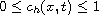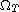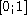Electron. J. Diff. Equ., Vol. 2012 (2012), No. 204, pp. 1-22.

### Numerical approximation for a degenerate parabolic-elliptic system modeling flows in porous media Rabah-Hacene Bellout

Abstract:
We present a numerical scheme for the approximation of the system of partial differential equations of the Peaceman model for the miscible displacement of one fluid by another in a two dimensional porous medium. In this scheme, the velocity-pressure equations are treated by a mixed finite element discretization using the Raviart-Thomas element, and the concentration equation is approximated by a finite volume discretization using the Upstream scheme, knowing that the Raviart-Thomas element gives good approximations for fluids velocities and that the Upstream scheme is well suited for convection dominated equations. We prove a maximum principle for our approximate concentration more preciselya.e. inas long as some grid conditions are satisfied - at the difference of Chainais and Droniou who have only observed that their approximate concentration remains in(and such is the case for other proposed numerical methods; e.g., [21,22]. Moreover our grid conditions are satisfied even with very large time steps and spatial steps. Finally we prove the consistency of the proposed scheme and thus are assured of convergence. A numerical test is reported.

Submitted August 1, 2012. Published November 24, 2012.
Math Subject Classifications: 76S05, 35K65, 35B65, 65M06.
Key Words: Mixed finite element methods; finite volume methods; porous media.Rabah-Hacene Bellout Faculté de Mathématiques, Université des Sciences et Technologies Houari Boumediene, Algiers, Algeria email: rbellout@usthb.dz